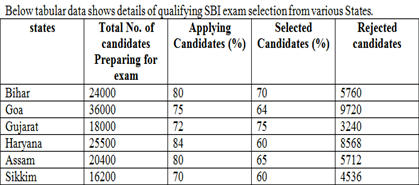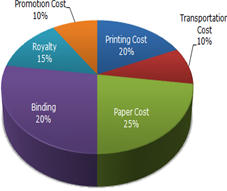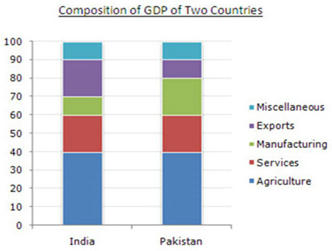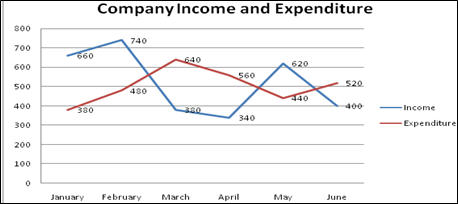## Magazine

English

### Tip of the Month

Tricks to solve Data Interpretation-

• Data Interpretation is one of the important topics asked in Banking Exams. Some aspirants avoid this section but they should always remember that DI is not a test of data calculation. Though some questions need a bit of calculation, others can be solved without calculation. If you can grasp it, it’s one of the most scoring sections and you can score a lot of marks here.
• Data Interpretation or DI refers to the implementation of procedures through which data is reviewed for the purpose of arriving at an inference.
• The word “Data” is based on facts and statistics collected together for reference or analysis. Data in numerical format helps us to draw conclusions by comparing the data.
• The word “Interpretation” is the act of explaining, re-framing or otherwise showing your own understanding of something.
• “Data Interpretation” is an act of analyzing data with the objective to gain useful information from it. It is done to draw conclusions from the given data. Different statistical tools are used to represent the data in organized structures
• DI is the art of decoding information presented in tables, bar graphs, pie charts and line graphs.

Some Tips and Tricks:

• Read the question carefully and analyze the Data carefully
• Skip questions if they are time-consuming
• Skip unnecessary calculations
• Pay close attention to the units used
• Use Approximation in Calculations
• Don’t use Calculator while Practice
• Don’t assume anything and plan the time allotment
• Patience and Persistence
• Familiarizing with different kinds of charts
• Enhancing the mental math skills
• Bucking up with the speed would thus help in saving time
• Regularly solving DI sets that require reasoning skills and logic and increasing your familiarity with such sets would help you sail through
• Rejection of DI sets based on the volume of data is a complete NO-NO. More data does not necessarily mean a more difficult set and vice versa.

Some concepts required to solve DI questions:

• Average = Sum of all the values/no. of values
• Percentage –
• a is what percentage of b = a/b x 100
• a is what percentage more or less than b = (a-b)/bx100
• Percentage change = (Final value – Initial value) / Initial value x 100
• Ratio = Comparison of like terms in its simplest terms.

Different types of sets of DI:

Tables- Tables are the most common types as most of the information can easily be represented in this form. A tabular form is a representation of data in a table format. It is easy and convenient to represent data in a tabular form.Pie-Chart- One of the most frequent ones in any test is a pie chart. Apart from calculation, they can be highly logical too. A pie chart is a circle which is drawn around an angle of 360 degrees.Bar graph- A bar graph is a way of representing data on the graph using X-axis and Y-axis. It is the most convenient way to present data.Line graph- The line graphs are pretty similar to the Bar Diagrams. It is simple to draw conclusions from Line Graph.